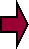SOCIETAL BEHAVIORAL PARAMETRICS
(W)

 (1W) Common first-world levels and collective behavior [Given: all (T) & (R) are P1 for (B)] Ascending societal levels Collective (P1, P2, and P3) domestic and foreign relations behavior 1W1 .htmlSurvival....................... [P1 = (T1) = R1- = B11] = Build families and churches [P2 = (T2) = R2+ = B21] = Use deceit and violence to get food/shelter [P3 = (T3) = R3+ = B31] = Be jailed or executed for murder 1W2 .htmlEconomic Security........[P1 = (T1) = R1- = B12] = Build education, business, & infrastructure [P2 = (T2) = R2 = B22] = Hoard resources greedily [P3 = (T3) = R3 = B32] = Be jailed for stealing 1W3 .htmlLove and Affection.......[P1 = (T2) = R2 = B23] = Use the government to tax others’ earnings [P2 = (T1) = R1- = B13] = Build hospitals, orphanages, & (T1) missions in (T2) & (T3) regions [P3 = (T3) = R3 = B33] = Be jailed for rape 1W4 .htmlStatus and Self-esteem..[P1 = (T2) = R2- = B24] = Use the government to force (T2) beliefs [P2 = (T3) = R3 = B34] = Enslave others [P3 = (T1) = R1- = B14] = Be jailed and enslaved for R1- behavior 1W5 .htmlSelf-actualization..........[P1 = (T3) = R3- = B35] = Suffer death from homicide, suicide, infanticide, disease & addiction [P2 = (T2) = R2- = B25] = Experience self-mutilation, individual & government bankruptcy [P3 = (T1) = R1- = B15] = Be murdered for (T1) beliefs

E1(2W) Common second-world levels and collective behavior [Given: all (T) & (R) are P1 for (B)] Ascending societal levels Collective (P1, P2, and P3) domestic and foreign relations behavior 2W1 .htmlSurvival.....................[P1 = (T2) = R2 = B21] = Use government deceit and violence to achieve [P2 = (T3) = R3 = B31] = Be jailed or executed for murder .html[P3 = (T1) = R1- = B11] = Be jailed or murdered for building churches 2W2 .htmlEconomic Security.....[P1 = (T3) = R3- = B32] = Use government to enslave others .html[P2 = (T2) = R2- = B22] = Hoard resources .html[P3 = (T1) = R1- = B12] = Be murdered for educating others in (T1) beliefs and have businesses confiscated 2W3, 2W4 and 2W5 are not achievable collectively

E2(3W) Common third-world levels and collective behavior [Given: all (T) & (R) are P1 for (B)] Ascending societal levels Collective (P1, P2, and P3) domestic and foreign relations behavior 3W1.html Survival......................[P1 = (T3) = R3- = B31] = Deceive, murder and enslave others [P2 = (T2) = R2- = B21] = Obtain food and shelter violently [P3 = (T1) = R1- = B11] = Be murdered and enslaved for holding (T1) beliefs 3W2, 3W3, 3W4 and 3W5 are not achievable collectively

E3